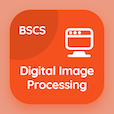Computer Science Courses Online

Digital Image Processing Certification Exam Tests

Digital Image Processing Practice Test 34

# Sampling and Fourier Transform of Sampled Function MCQ Questions PDF - 34

The Book Sampling and Fourier Transform of Sampled Function Multiple Choice Questions and Answers (MCQs), sampling and fourier transform of sampled function MCQs Quiz PDF download chapter 3-34 to study online digital image processing course. Study Filtering in Frequency Domain quiz answers PDF, sampling and fourier transform of sampled function Multiple Choice Questions (MCQ Quiz) for online college degrees. The eBook Sampling and Fourier Transform of Sampled Function MCQs App Download: sampling and fourier transform of sampled function, line detection in image segmentation, point line and edge detection, spatial and intensity resolution, background of intensity transformation test prep for computer and information science.

The MCQs: The band limited function can be recovered from its samples if the acquired samples are at rate twice the highest frequency, this theorem is called PDF, "Sampling and Fourier Transform of Sampled Function" App (Android & iOS) Free with sampling theorem, sampling theorem, sampling theorem, and sampling theorem choices for accelerated computer science degree online. Practice filtering in frequency domain questions and answers, Google eBook to download free sample for bachelor's degree in computer science.

## Image Processing MCQ: Sampling & Fourier Transform of Sampled Function Quiz - 34

MCQ: The band limited function can be recovered from its samples if the acquired samples are at rate twice the highest frequency, this theorem is called

A) sampling theorem
B) sampling theorem
C) sampling theorem
D) sampling theorem

MCQ: The preferred direction of mask is weighted with the

A) low value coefficients
B) high value coefficients
C) mid value coefficients
D) double value coefficients

MCQ: Points other than exceeding the threshold in output image are marked as

A) 0
B) 1
C) 11
D) x

MCQ: Type of zooms are

A) 8
B) 6
C) 4
D) 2

MCQ: In the formula g(x,y) = T[ƒ(x,y)], T is the

A) transformed image
B) transformation vector
C) transformation theorem
D) transformation function

### Mock Tests: Digital Image Processing Course Prep

Download Digital Image Processing Quiz App, Database Management System MCQ App, and Computer Fundamentals MCQs App to install for Android & iOS devices. These Apps include complete analytics of real time attempts with interactive assessments. Download Play Store & App Store Apps & Enjoy 100% functionality with subscriptions!